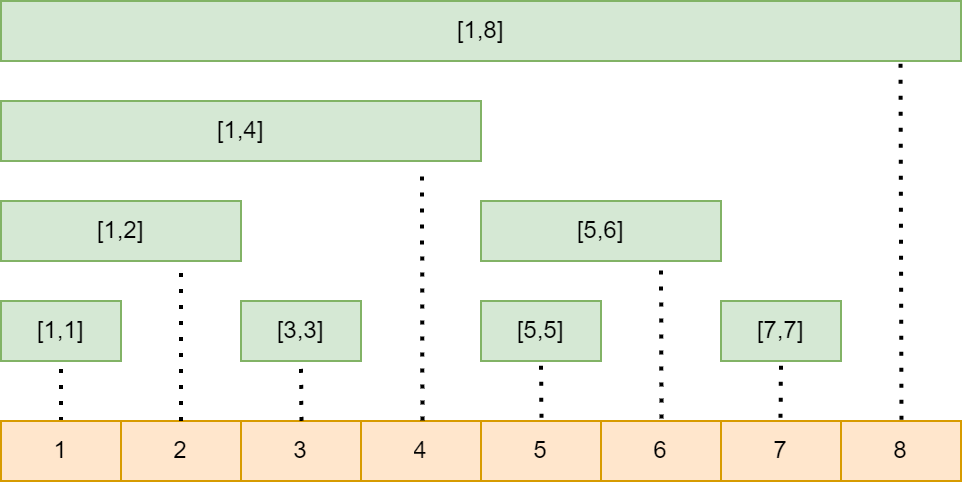# Binary Indexed (Fenwick) Tree

Into to Fenwick Tree aka Binary Indexed Tree
🔗

Binary Indexed tree, also called as Fenwick tree is a type of data structure used to efficiently query for range sums on an array of values. Range sums / prefix sums are the totals of all the numbers between a certain start and end points in an array/list.

## The Problem

Normally if we wish to compute sum of all numbers from indexes 0 to n in an array, we would go through each element starting from index-0 and keep adding all the elements until index-n. This takes O(n) time and is not efficient when the size is very large and we need to perform a lot of queries.

One solution to this is to use prefix sum array. A prefix sum array is a pre-computed array where the value at any index is equal to the sum of all the numbers from 0 to this index in the original array. If we receive a query which asks us to get the total sum of indexes between x and y, we need to subtract the prefix sum at index x-1 from the prefix sum at index y, which gives us the sum of elements from indexes x to y. This takes only 2 operations and hence we could retrieve the range sum in O(1) time. But the catch is, if suppose a value at index `i` changes, all the prefix sums for indexes greater `i` will change and this takes O(N) time.

To summarize, the first approach takes `O(N)` time to compute sum of all elements between any given indexes but it takes `O(1)` time to update any element. In the second approach using prefix sums, it takes `O(1)` time to compute sum of all elements between any given indexes but it takes `O(N)` time to update the prefix sum array if any element in the original array changes.

The question now is can we have a balance between both? i.e, have a reasonable time complexity at the time of updating any element while also having a reasonable time complexity for computing prefix sums? Binary Indexed Tree or Fenwick Tree is one solution to this question.

## The Intuition behind Fenwick Tree

Any given number can be split into multiple numbers which are powers of 2. This is how binary system works. Below are examples of how the numbers can be re-written in sums of powers of two:

``````14 = 2^3 + 2^2 + 2^1
13 = 2^3 + 2^2 + 2^0
12 = 2^3 + 2^2
...
``````

This way, maximum number of divisions that need to be made is `log(N)`. Similar to this concept, the sum until any index can be split into multiple segments with lengths in multiples of `2` and add up all the segments to compute prefix sum at any index.

Below is the illustration the above concept (Inspired from a image from this article).